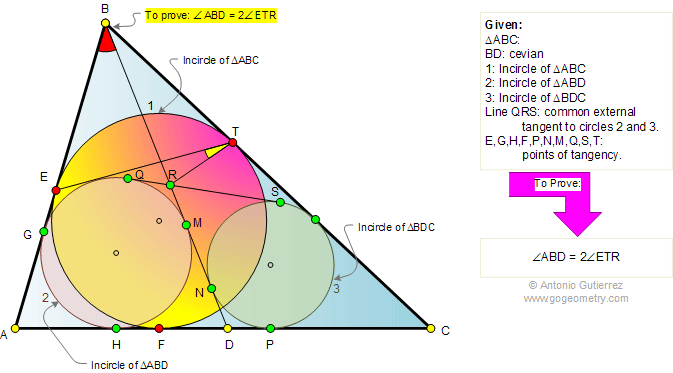Problem 350. Triangle, Cevian, Incircles, Tangents, Tangency Points, Angles. Level: High School, College, SAT Prep. The figure shows a triangle ABC with point D on side AC. Circles 1, 2, and 3 are the incircles of triangles ABC, ABD, and BDC, respectively. QS is the common tangent to circles 2 and 3. QS intersects to cevian BD at R. If E,G,H,F,P,N,M,Q,S, and T are points of tangency, prove that the angle ABD is double the angle ETR.Recent Additions